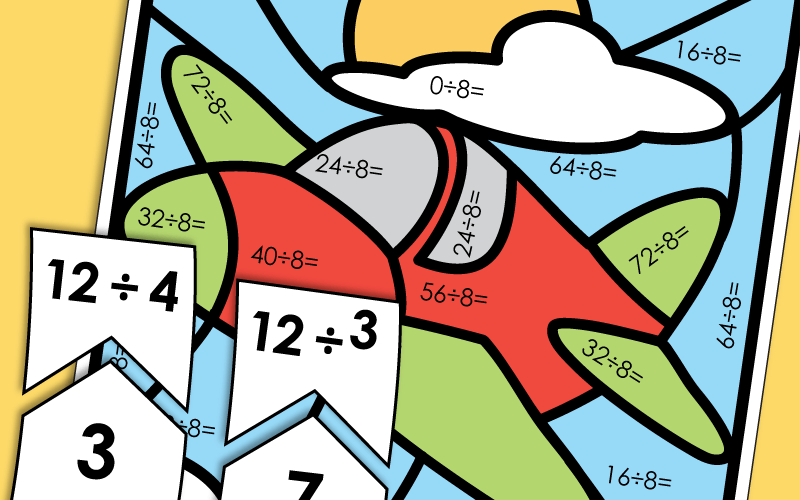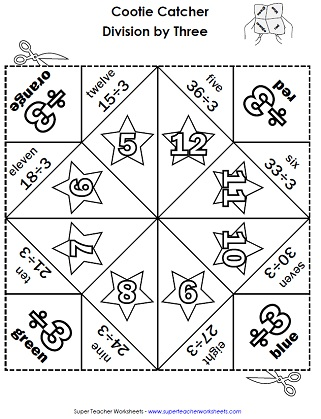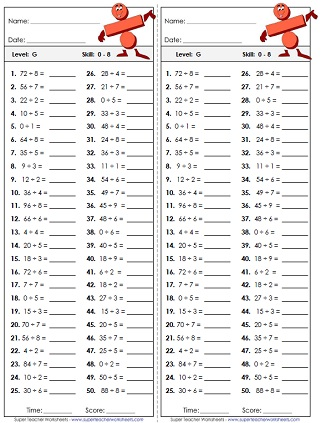# Basic Division Worksheets

Printable worksheets with basic division facts.  Includes dividends up to 81 and divisors up to 9, as well as basic division with remainders.## Games

Cut apart the stars. Match division problems with their quotients to make stars. (Note: There is also a full-color version of this file. To access the color version, click the "alt" link below.)
Play this fun game with your class to practice basic division and multiplication.
Match the division facts with the correct quotient in this memory card game.
Practice basic division facts using these printable puzzle pieces.
In this game, students take turns rolling a die. The number they roll corresponds with a column of basic division problems that they have to read and solve as quickly as possible.
Hunt for hidden division facts with this printable number search puzzle. Find all 20 division facts, and write the complete number sentences on your answer key.
Print out this worksheet with caterpillars containing division math problems. Have your students solve the problems and write their answers in the space provided.

## Worksheets

Students draw objects and divide them into equal groups.  A great way to begin teaching the concept of division.
Same worksheet as above, but the numbers and objects are different.
Divide the objects into equal groups to find the answers to basic division facts.
What has four wheels and flies? To answer the riddle, kids will solve a series of basic division problems. Then match the letters to the answers at the bottom of the page.
Print out this page so your students can practice division facts on this cut and glue worksheet.
Find the quotients of these basic division problems with divisors up to 12.
Multiplication-division fact families; Give three numbers, students write 4 basic facts
Picture clues will help students solve these basic division word problems.
Twenty-five basic division problems.  Includes two word problems.  Superhero theme.
Answer the division thinking questions. This worksheet includes some short answer questions. Skills include identifying the quotient, dividend, and divisor, as well as explaining the relationship between multiplication and division.
Why do sharks only swim in saltwater? To decode the answer to the riddle, students must find the missing dividend for each division problem.
This worksheet focuses on the zero property of division and the identity property of division.
Can you find all of the division facts hidden in this puzzle?
Find each quotient and write X or O over the corresponding number on the tic-tac-toe boards.
For this activity, students will use a cryptogram key to translate picture symbols into basic division problems. Then they will write the quotient for each.

## Division with Arrays

Use the array illustrations to find and solve basic division facts.
Answer the questions for each array, and find the division fact for each.
Write the fact family shown by each array. Write all four facts for each illustration.

## Timed Quizzes

Test your students' division skills with this timed quiz with divisors of 1s and 2s, as well as dividends of 0.  Recommended time: 5 minutes.
The problems on this fifty question test have divisors 1s, 2s, and 3s, and dividends of 0.  Recommended time: 5 minutes.
The problems on this fifty question test have divisors 1s, 2s, and 3s, 4s, and dividends of 0.  Recommended time: 5 minutes.
The problems on this fifty question test have divisors 1s, 2s, and 3s, 4s, 5s, and dividends of 0.  Recommended time: 5 minutes.
The problems on this fifty question test have divisors 1s, 2s, and 3s, 4s, 5s, 6s, and dividends of 0.  Recommended time: 5 minutes.
The problems on this fifty question test have divisors 1s, 2s, and 3s, 4s, 5s, 6s, 7s, and dividends of 0.  Recommended time: 5 minutes.
The problems on this fifty question test have divisors 1s, 2s, and 3s, 4s, 5s, 6s, 7s, 8s, and dividends of 0.  Recommended time: 5 minutes.
The problems on this fifty question test have divisors 1s, 2s, and 3s, 4s, 5s, 6s, 7s, 8s, 9s, and dividends of 0.  Recommended time: 5 minutes.
The problems on this fifty question test have divisors 1s, 2s, and 3s, 4s, 5s, 6s, 7s, 8s, 9s, 10s, and dividends of 0.  Recommended time: 5 minutes.
The problems on this fifty question test have divisors 1s, 2s, and 3s, 4s, 5s, 6s, 7s, 8s, 9s, 10s, 11s, and dividends of 0.  Recommended time: 5 minutes.
The problems on this fifty question test have divisors 1s, 2s, and 3s, 4s, 5s, 6s, 7s, 8s, 9s, 10s, 11s, 12s, and dividends of 0.  Recommended time: 5 minutes.

## Basic DivisionWorksheet Generator

Basic Division Worksheet Generator

Create your own custom division printables with basic facts. You choose the range for the subtrahends and the differences. This generator tool lets you to create worksheets with 25 or 50 problems. Choose whether or not you want remainders.

## Mystery Pictures

Solve the basic division facts. All problems have dividends of 81 or less. Color the mystery scene according to the key to see a pail and colorful beach ball.
Color the page based on the answers to the division problems and the key at the bottom of the page. The patterns on the two worksheets look the same, but depending on which you complete you may reveal either a farm or a lighthouse.
Find the quotients and color the picture according to the key to reveal a clownfish.  Divisors up to 9.
Students solve basic division problems to color in the picture of a fox using the key.
The hot air balloon mystery math picture requires students solve basic division with divisors up to 9.
Find the quotient for each basic division fact. Then color to reveal a ladybug on a flower.
Write the quotient for each division problem, then color according to the key. (Note: This worksheet requires three different shades of brown crayons.)
Write the quotients, then color the mystery picture to reveal a parrot.
This file includes two mystery pictures. They look the same, but one worksheet will result in a boat and the other will result in a plane.
Using the key based on quotients, color the robot picture after solving the division problems.
More division problems with divisors up to 9.  Mystery picture makes a rocket ship.
Find the quotients of these basic division problems and color according to the key.  Divisors up to 9.
Solve all of the division problems on this worksheet. Use the answers to find out which colors to use when coloring in this back-to-school-themed picture.
Students will solve all of the basic division problems and then color in the spaces according to the key at the bottom to reveal colorful sea shells at the beach.
Solve to find the quotients for each simple division problem. Then color the friendly sea monster picture according to the key at the bottom of the worksheet.
Solve the division problems and color accordingly to make the mystery pictures appear.
Find the quotients and color to reveal two different summer-themed mystery pictures!
Find the quotients to reveal a picture of a black and brown spotted canine.
Sailboat mystery picture in which all facts require students to divide by 4.
To see the giant blue whale, students will have to find the quotients to the basic division problems. All problems have a divisor of 6.
Division facts (6s only; Missing dividends) mystery picture of fruits ad vegetables, including guava fruit, apple, carrot, and banana.
Solve the division facts (7s only) and color to reveal a castle.
Each of the division facts on this worksheet has eight as a divisor. Solve and color to see a neat airplane picture.

## Origami Fortune Tellers(aka Cootie Catchers)

Fold the origami fortune teller and use it to practice the toughest basic division facts. Facts include twenty-eight divided by four, thirty-two divided by eight, and sixty-three divided by seven.
Cut, fold, and color this origami cootie catcher (aka fortune teller) game. Players review division facts with two.
(Up to 24 divided by 2)
Players practice dividing by three with this fun math game.
(Up to 36 divided by 3)
Create and play with this paper fortune teller, which includes basic division facts with four.
(Up to 48 divided by 4)
Your students will enjoy practicing their basic division facts with this fun cootie catcher.
(Up to 60 divided by 5)
Learn to divide by six with this terrific paper fortune teller math game.
(Up to 72 divided by 6)
Do your students know their basic division facts with sevens? If not, give them this fun printable game to review their basic facts.
(Up to 84 divided by 7)
Review simple division facts with eight. Students color and fold this cootie catcher, then play a division review game with friends.
(Up to 96 divided by 8)
Practice dividing numbers by nine with this entertaining, foldable fortune teller game.
(Up to 108 divided by 9)
Dividing by ten is lots of fun with this printable division game worksheet.
(Up to 110 divided by 10)
Kids will love using origami cootie catchers to learn their division facts.
(Up to 121 divided by 11)
Practice those tricky twelves with this division fortune teller game.
(Up to 144 divided by 12)

## Basic Remainders

Solve basic division problems with remainders. Then use the answers to find the answer to the riddle.
This file has thirty task cards. Use them for learning centers, math games, exit slips, or extra practice.
Decode the picture symbols and change them into division problems using the key. Then solve.

## Flashcards

Complete set of division flash cards, up to 144 / 12.
Practice basic division facts with these triangular fact family cards.
Print these small flashcards on card stock to help students practice their basic division facts. (Up to 144 / 12.)
Averages Worksheets

Find the average, or mean, or the numbers.

Fact Families (Basic)

Print basic division and multiplication number bond and fact family worksheets.

Long Division

Divide larger numbers with 2, 3, or 4 digit dividends.

## Sample Images ofDivision WorksheetsMy Account
Site Information